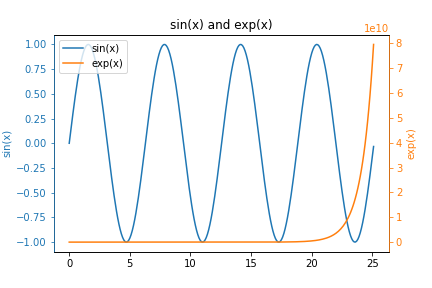## Calculating Vacancy Concentration with PythonIn this post, we will complete a problem that might come up in an introductory Materials Science and Engineering class. We'll calculate the number of vacancy defects in a material using Python.

The following is a calculation of the number of vacancy defects in a material. All crystalline and poly-crystalline …

## How to make y-y plots with MatplotlibIn this post, you will learn how to create y-y plots with Python and Matplotlib. y-y plots are a type of line plot where one line corresponds to one y-axis and another line on the same plot corresponds to a different y-axis. y-y plots typically have one vertical y-axis on …

## Hear my story about deploying JupyterHub on the Running in Production PodcastI was a guest on the Running in Production podcast with Nick Janetakis! We talked about how a Community College professor deployed JupyterHub online using a Digital Ocean cloud server. Check out the podcast episode here.

## Deploy a Jupyter Notebook Online with Voila and HerokuJupyter notebooks are a great way to write and run Python code. Jupyter notebooks can produce text output, plots, and animations. Static Jupyter notebooks can be shared on GitHub.com and nbviewer. Another way to share Jupyter notebooks is a great Python package called Voila. Voila turns Jupyter notebooks into …

## Estimating the Deflection of a Truncated Cone using PythonIn this post, we will complete a problem that might come up in a Strength of Materials class. We'll estimate the deflection of a truncated cone given an applied load using Python.

### The Problem¶

Below is an engineering mechanics problem that can be solved in Python. Follow along this post …